Finding point when tangent is parallel/ perpendicular

Chapter 6 Class 12 Application of Derivatives
Concept wise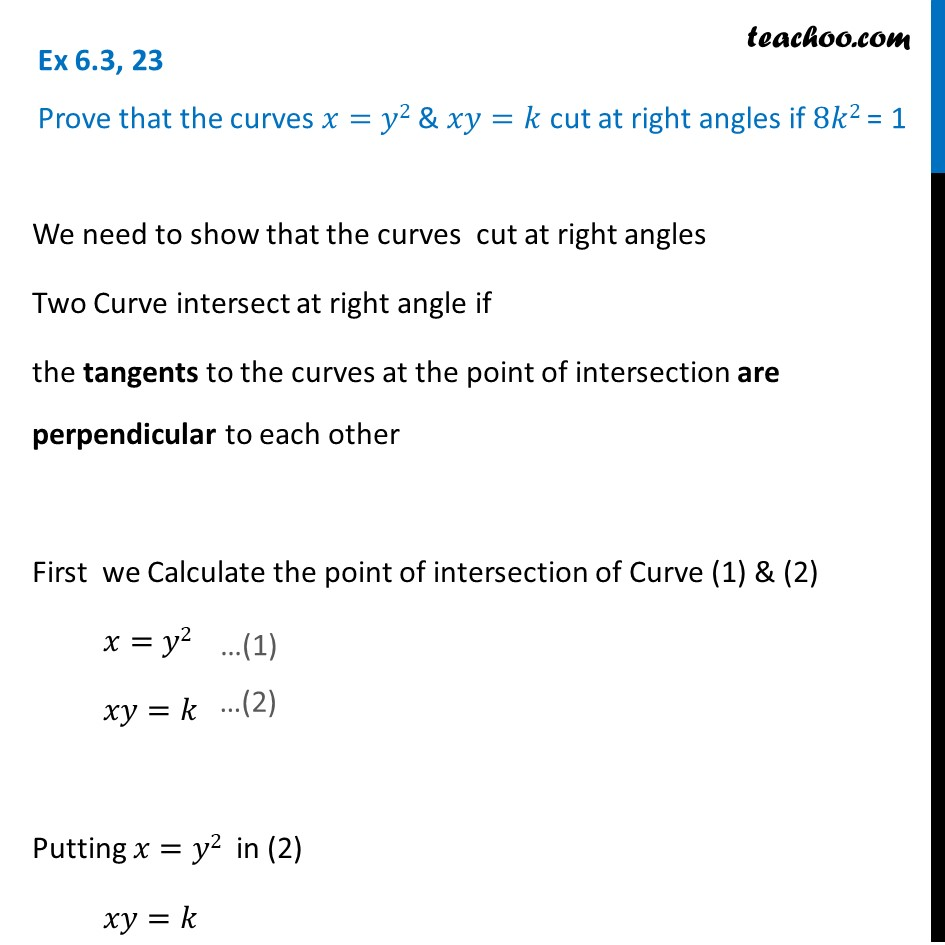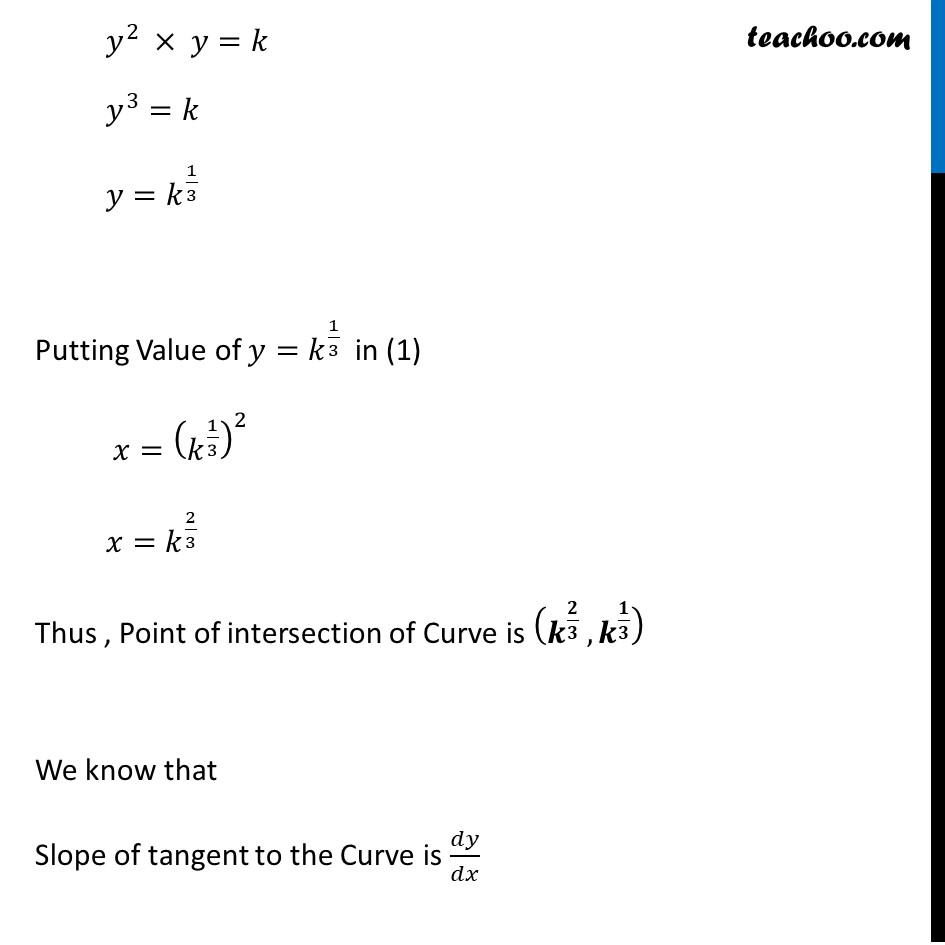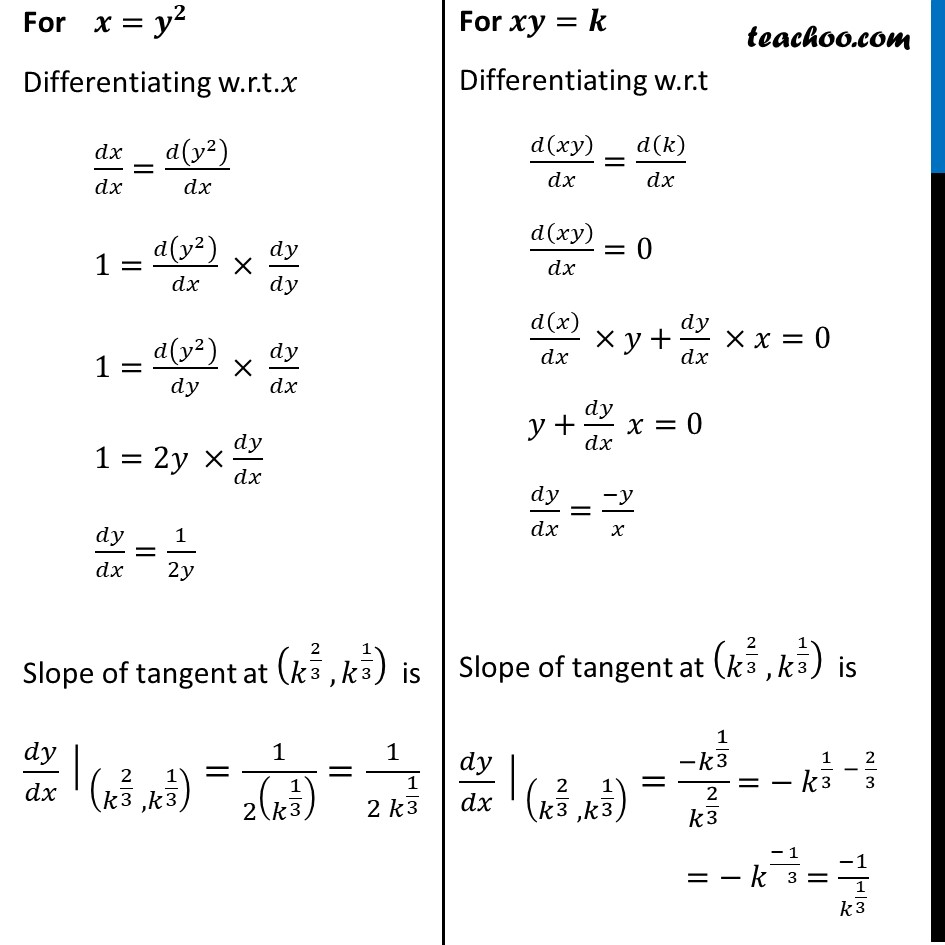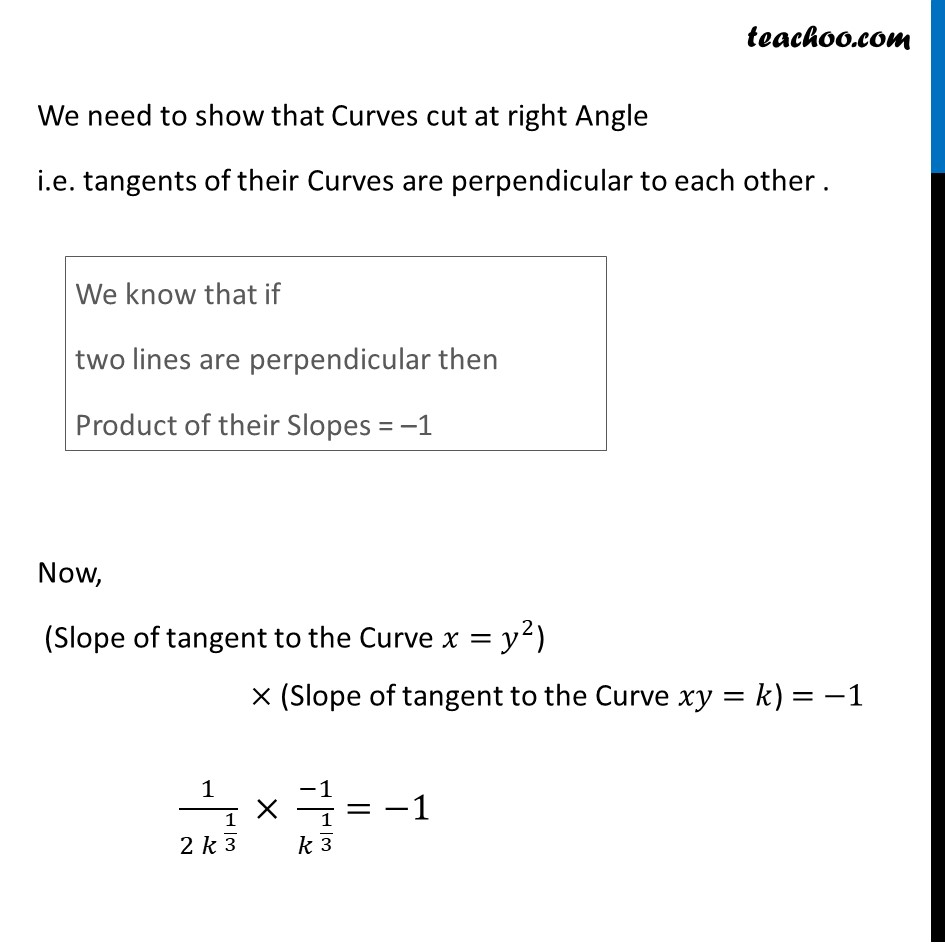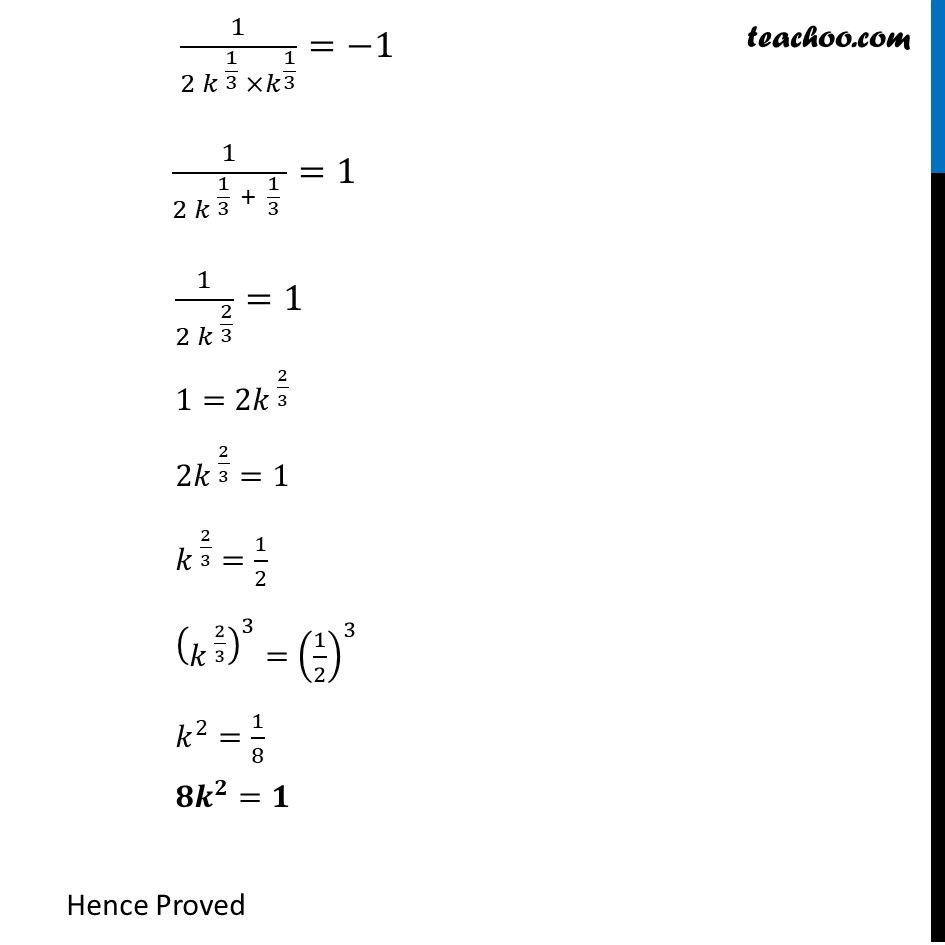Introducing your new favourite teacher - Teachoo Black, at only ₹83 per month

### Transcript

Ex 6.3, 23 Prove that the curves 𝑥=𝑦2 & 𝑥𝑦=𝑘 cut at right angles if 8𝑘2 = 1We need to show that the curves cut at right angles Two Curve intersect at right angle if the tangents to the curves at the point of intersection are perpendicular to each other First we Calculate the point of intersection of Curve (1) & (2) 𝑥=𝑦2 𝑥𝑦=𝑘 Putting 𝑥=𝑦2 in (2) 𝑥𝑦=𝑘 𝑦^2 × 𝑦=𝑘 𝑦^3=𝑘 𝑦=𝑘^(1/3) Putting Value of 𝑦=𝑘^(1/3) in (1) 𝑥=(𝑘^(1/3) )^2 𝑥=𝑘^(2/3) Thus , Point of intersection of Curve is (𝒌^(𝟐/𝟑) ,𝒌^(𝟏/𝟑) ) We know that Slope of tangent to the Curve is 𝑑𝑦/𝑑𝑥 For 𝒙=𝒚^𝟐 Differentiating w.r.t.𝑥 𝑑𝑥/𝑑𝑥=𝑑(𝑦^2 )/𝑑𝑥 1=𝑑(𝑦^2 )/𝑑𝑥 × 𝑑𝑦/𝑑𝑦 1=𝑑(𝑦^2 )/𝑑𝑦 × 𝑑𝑦/𝑑𝑥 1=2𝑦 ×𝑑𝑦/𝑑𝑥 𝑑𝑦/𝑑𝑥=1/2𝑦 Slope of tangent at (𝑘^(2/3) , 𝑘^(1/3) ) is 〖𝑑𝑦/𝑑𝑥│〗_((𝑘^(2/3) , 𝑘^(1/3) ) )=1/2(𝑘^(1/3) ) =1/(2 𝑘^(1/3) ) For 𝒙𝒚=𝒌 Differentiating w.r.t 𝑑(𝑥𝑦)/𝑑𝑥=𝑑(𝑘)/𝑑𝑥 𝑑(𝑥𝑦)/𝑑𝑥=0 𝑑(𝑥)/𝑑𝑥 ×𝑦+𝑑𝑦/𝑑𝑥 ×𝑥=0 𝑦+𝑑𝑦/𝑑𝑥 𝑥=0 𝑑𝑦/𝑑𝑥=(−𝑦)/𝑥 Slope of tangent at (𝑘^(2/3) , 𝑘^(1/3) ) is 〖𝑑𝑦/𝑑𝑥│〗_((𝑘^(2/3) , 𝑘^(1/3) ) )=(−𝑘^(1/3))/𝑘^(2/3) =−〖 𝑘〗^(1/3 − 2/3) =−〖 𝑘〗^((− 1)/( 3) )=(−1)/𝑘^(1/3) We need to show that Curves cut at right Angle i.e. tangents of their Curves are perpendicular to each other . Now, (Slope of tangent to the Curve 𝑥=𝑦^2) × (Slope of tangent to the Curve 𝑥𝑦=𝑘) =−1 1/(2 𝑘^( 1/3) ) × (−1)/𝑘^( 1/3) =−1 We know that if two lines are perpendicular then Product of their Slopes = –1 1/(2 〖𝑘 〗^(1/3) ×𝑘^(1/3) )=−1 1/(2 𝑘^( 1/3 + 1/3) )=1 1/(2 𝑘^( 2/3) )=1 1=2𝑘^( 2/3) 2𝑘^( 2/3)=1 𝑘^( 2/3)=1/2 (𝑘^( 2/3) )^3=(1/2)^3 𝑘^2=1/8 〖𝟖𝒌〗^𝟐=𝟏 Hence Proved